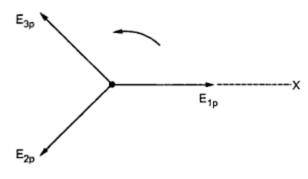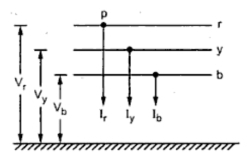### Fault Current Calculation using Symmetrical Components

Protective Relaying (Part10)
In case of three phase balanced system, currents and voltages are equal in magnitude and are displaced from each other by 120o. The currents and voltages are thus said to be symmetrical. The analysis of such system is easy which is done on per phase basis. But when the load is unbalanced, the analysis using normal techniques becomes difficult. Under such case, method of symmetrical components can be adopted as suggested by Fortescue. According to this theorem, the unbalanced phasors can be resolved into three balanced systems of phasors. The balanced sets of component can be given as (a) positive sequence component (b) negative sequence component (c) zero sequence component.
A system of vectors is having positive phase sequence when it has all vectors having same magnitude and are displace by 120o. They have same time interval to achieve fixed axis of reference as that of generated voltage. the positive sequence is designated by use of subscript P. It is shown in the Fig. 1. The vectors come to X axis in order to 1, 2 and 3.Fig.1  Positive phase sequence with anticlockwise rotation of vectors (1-2-3)
A system of vector is having negative phase sequence when it has all vectors having same magnitude and are displaced by . But arrive at the reference axis at a regular interval same as that of positive sequence but in reverse order i.e. in order of 1, 3, 2. It is shown in the Fig. 2 suffix n is used to designate negative sequence.Fig.2  Negative phase sequence with anticlockwise rotation of vectors (1-2-3)
A system vectors is having zero phase sequence if all the three vectors are not displaced in time from each other and there will be no phase sequence. In such cases the current or voltage in the 3 phase circuit will vary simultaneously in all the 3 phases. Such a phase sequence is shown in the Fig. 2(a) suffix 0 is used to designate zero sequence.Fig. 2 (a) Zero phase sequence
Consider a system having three vectors and it can be resolved into positive, negative and zero phase sequence components as shown in the Fig. 3. It is possible to get 3 vectors by using following equations.
E1 = E1P  + E1n  + E10
E2 = E2P  + E2n  + E20
E3 = E3P  + E3n + E30Fig. 3
Consider in general a power system network as shown in the Fig. 4 suppose that fault occurs at point P in this system. Due to this currents Ir, Iy, Ib flow out of the system while Vr, Vy, Vb are the line voltages with respect to ground.Fig. 4

If it is assumed that the system is operating at no load before occurring a fault, then the positive sequence voltages of all synchronous machines will be equal and has value same as prefault voltage at P. Let this voltage be Er.
The voltage Er is present only in the positive sequence network. There is no coupling between sequence network, the sequence voltages at P can be given in terms of sequence currents and Thevenin sequence impedance as

Based on the types of fault, the sequence voltages and currents constrained resulting to a particular connection of sequence networks. The sequence currents and voltages and fault currents and fault voltages can then be easily obtained.

1.2.